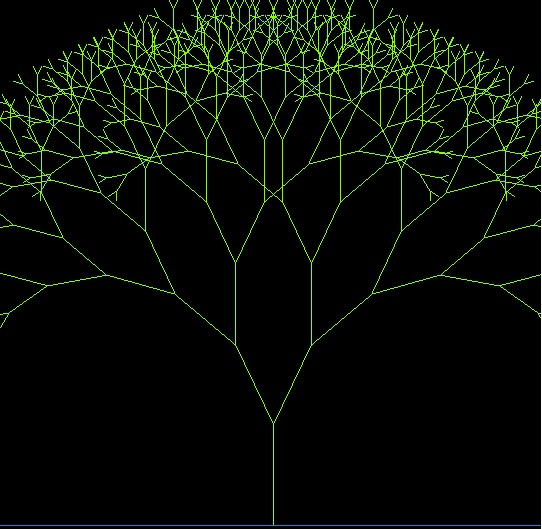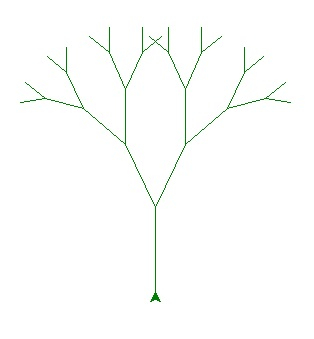# Fractal Trees in Python

PythonServer Side ProgrammingProgramming

Fractal patterns are all around us in nature. Like a small branch taken out of the leaf of a fern leaf resembles the leaf itself. Or a pebble often resembles the shape of a mountain! So this idea of a repetition of small pattern to generate a large pattern is known as a fractal tree. In python programming we can also generate fractal trees by using various modules available.

## Using pygame Module

This module provides us with the required functions to generate the fractal trees. Here we first defines the screen layout size and then define the deepness up to which the patterns will repeat themselves. Recursion has a big role here as we are going to repeat the same pattern again and again up to a certain depth.

### Example

import pygame, math

pygame.init()
screen = pygame.display.set_mode((750, 650))
pygame.display.set_caption("Fractal Tree")
display = pygame.display.get_surface()

def drawTree(a, b, pos, deepness):
if deepness:
c = a + int(math.cos(math.radians(pos)) * deepness * 10.0)
d = b + int(math.sin(math.radians(pos)) * deepness * 10.0)
pygame.draw.line(display, (127,255,0), (a, b), (c, d), 1)
drawTree(c, d, pos - 25, deepness - 1)
drawTree(c, d, pos + 25, deepness- 1)

def process(event):
if event.type == pygame.QUIT:
exit(0)

drawTree(370, 650, -90, 10)
pygame.display.flip()
while True:
process(pygame.event.wait())

### Output

Running the above code gives us the following result:## Using Turtle

Using turtle module we can follow a similar approach. Here the turtle program starts drawing the branches of the tree as repeated pattern by just changing the direction of the drawing. We define the angles at which the function will repeat itself and then we get the complete tree.

### Example

import turtle
def tree(Length,n):
if Length > 10:
n.forward(Length)
n.right(25)
tree(Length-15,n)
n.left(50)
tree(Length-15,n)
n.right(25)
n.backward(Length)

def function():
n = turtle.Turtle()
data = turtle.Screen()
n.left(90)
n.up()
n.backward(100)
n.down()
n.color("green")
tree(85,n)
data.exitonclick()

function()

### Output

Running the above code gives us the following result: